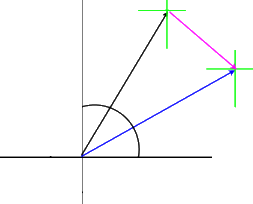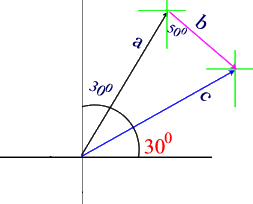#### Additing Vectors GraphicallyTake your time when using this technique.

It requires accuracy when scaling, measuring and drawing vectors.  Only if you're accurate you will obtain correct results.

Step-by-step approach:

1. Choose a scale according to the given information
2. Start with a set of reference axis and draw the first vector using a ruler to draw its magnitude to sale and a protractor to report its direction.
3. At the head of the vector draw a small cross.  This is a new reference axis to guide you in drawing successive vectors.
4. Draw the second vector (and the third if necessary) using steps 1 to 3.
5. Draw the resultant vector from the tail of the first vector to the head of the last vector.
6. Use a ruler and protractor to report the magnitude and direction of the resultant vector properly.

Example:

A pigeon flies 5 km [N300E], then 2.5 km [E400S]. Calculate its final displacement.

Solution:

Step 1 - choose an appropriate  a scale such as:
scale = 1cm = 1 km; therefore: a = 5 cm  [N300E], and =  2.5 cm [E400S].
Step 2 - draw vectors and b to scale
Step 3 - join the tail of vector a to the head of vector  b to find the resultant vector c.
Step 4 - measure resultant vector c using a ruler and convert to real-time units using your scale.
Step 5 - use a protractor to measure and report the direction of resultant vector c.
Step 6 - Conclusion - The final displacement  of the pigeon is c = 5.2 km [E300N]

Refer to the diagrams below:Figure 1 - Steps 1 to 4Figure 2 - Steps 5 & 6